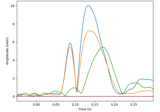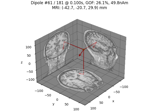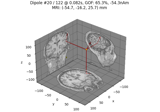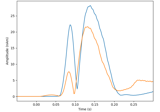# mne.viz.plot_dipole_amplitudes#

mne.viz.plot_dipole_amplitudes(dipoles, colors=None, show=True)[source]#

Plot the amplitude traces of a set of dipoles.

Parameters
dipoles`list` of instance of `Dipole`

The dipoles whose amplitudes should be shown.

colors

Color to plot with each dipole. If None default colors are used.

showbool

Show figure if True.

Returns
fig`matplotlib.figure.Figure`

The figure object containing the plot.

Notes

New in version 0.9.0.

## Examples using `mne.viz.plot_dipole_amplitudes`#Compute a sparse inverse solution using the Gamma-MAP empirical Bayesian method

Compute a sparse inverse solution using the Gamma-MAP empirical Bayesian methodCompute sparse inverse solution with mixed norm: MxNE and irMxNE

Compute sparse inverse solution with mixed norm: MxNE and irMxNECompute Rap-Music on evoked data

Compute Rap-Music on evoked dataCompute MxNE with time-frequency sparse prior

Compute MxNE with time-frequency sparse prior